# GSEB Solutions Class 12 Maths Chapter 7 Integrals Ex 7.11

Gujarat Board GSEB Textbook Solutions Class 12 Maths Chapter 7 Integrals Ex 7.11 Textbook Questions and Answers.

## Gujarat Board Textbook Solutions Class 11 Maths Chapter 7 Integrals Ex 7.11

By using properties of definite integrals, evaluate the following:
Question 1.
$$\int_{0}^{\frac{\pi}{2}}$$ cos2x
Solution: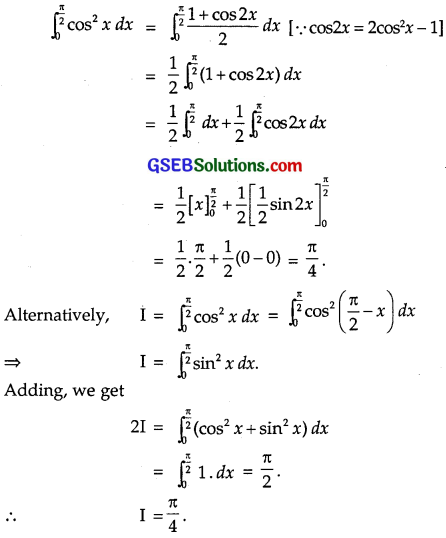Question 2.
$$\frac{\sqrt{\sin x}}{\sqrt{\sin x}+\sqrt{\cos x}}$$dx
Solution: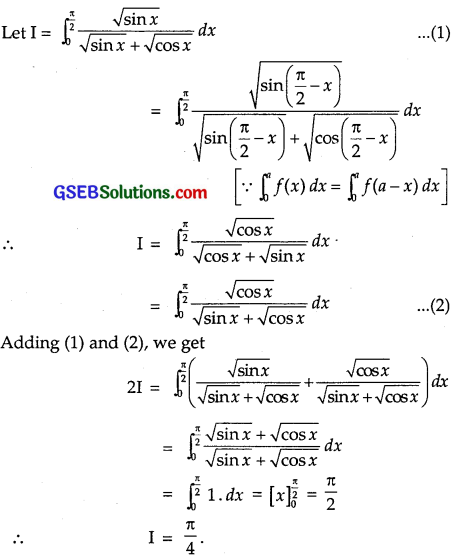Question 3.
$$\int_{0}^{\frac{\pi}{2}} \frac{\sin ^{\frac{3}{2}} x}{\sin ^{\frac{3}{2}} x+\cos ^{\frac{3}{2}} x}$$dx
Solution: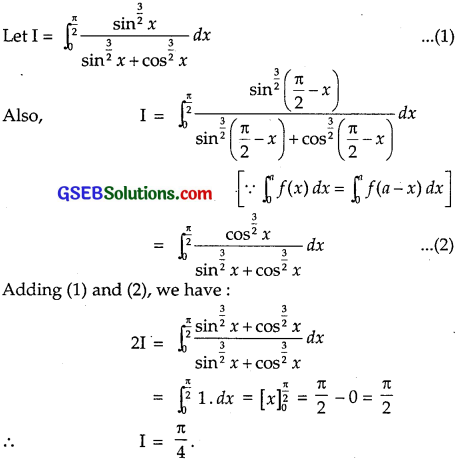Question 4.
$$\int_{0}^{\frac{\pi}{2}} \frac{\cos ^{5} x}{\sin ^{5} x+\cos ^{5} x}$$
Solution: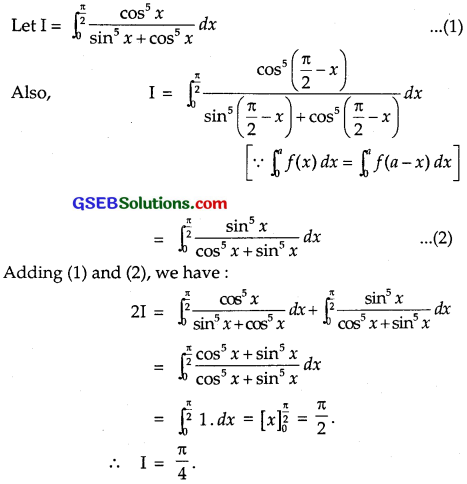Question 5.
$$\int_{-5}^{5}$$|x + 2|dx
Solution: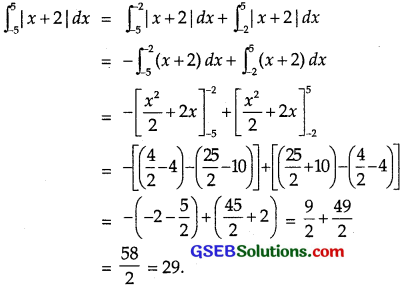Question 6.
$$\int_{2}^{8}$$|x – 5|dx
Solution: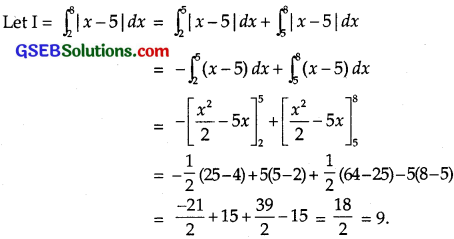Question 7.
$$\int_{0}^{1}$$x(1 – x)ndx
Solution: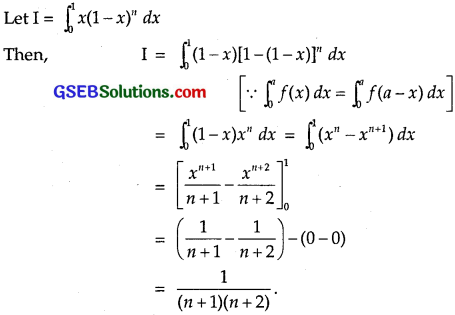Question 8.
$$\int_{0}^{\frac{\pi}{2}}$$log(1 + tan x)dx
Solution: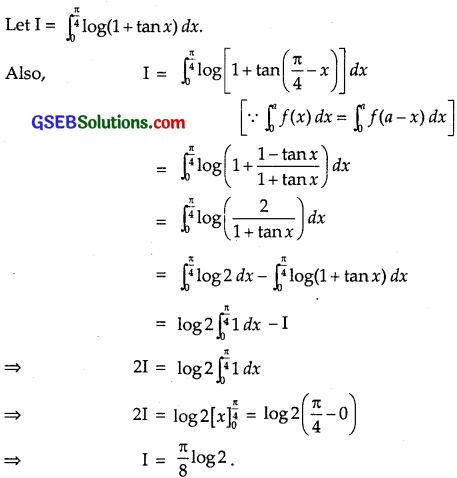Question 9.
$$\int_{0}^{2}$$x$$\sqrt{2-x}$$dx
Solution: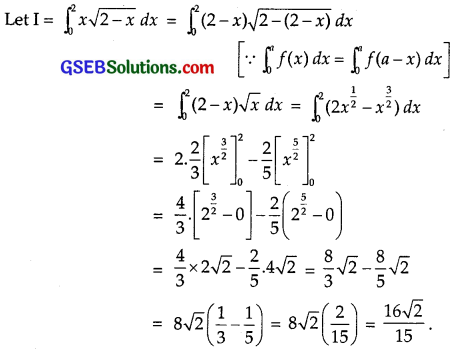Question 10.
$$\int_{0}^{\frac{\pi}{2}}$$(2log sin x – log sin2x)dx
Solution: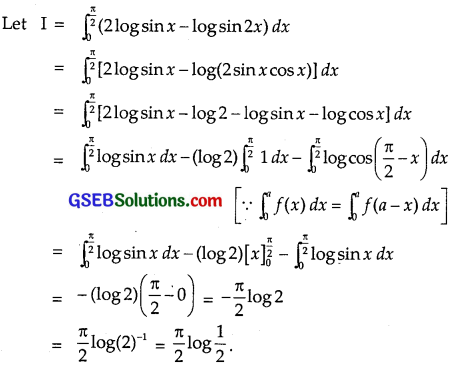Question 11.
$$\int_{-\frac{\pi}{2}}^{\frac{\pi}{2}} \sin ^{2} x d x$$
Solution:
Let f(x) = sin2x. Then,
f(- x) = [sin(- x)]2 = (- sin x)2 = sin2x = f(x).
∴ f(x) is an even function.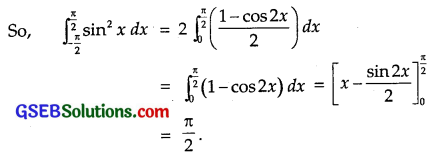Question 12.
$$\int_{0}^{π}$$ $$\frac{xdx}{1+sinx}$$
Solution: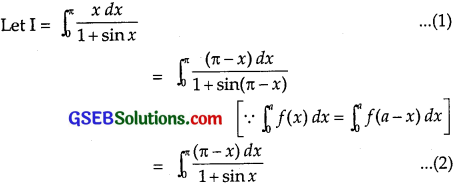Adding (1) and (2), we have: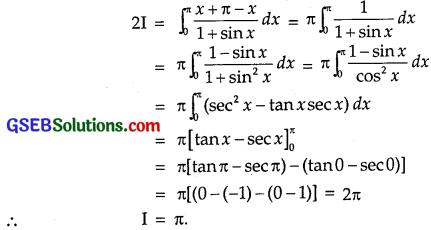Question 13.
$$\int_{-\frac{\pi}{2}}^{\frac{\pi}{2}} \sin ^{7} x d x$$
Solution:
Let f(x) = sin7x
f(- x) = [sin (- x)]7 = (- sin x)7 = – sin7x = – f(x)
⇒ f(x) is an odd function of x.
But $$\int_{-a}^{a}$$f(x) dx = 0 when x is odd.
∴ $$\int_{\frac{\pi}{2}}^{\frac{\pi}{2}} \sin ^{7} x$$ dx = 0.Question 14.
$$\int_{0}^{2π}$$ cos5x
Solution:
f(x) = cos5x
∴ f(2π – x) = cos5(2π – x) = cos5x = f(x).
∴ I = $$\int_{0}^{2π}$$ cos5x dx = 2$$\int_{0}^{π}$$cos5x dx.
Again taking g(x) = cos5x, we get
g(π – x) = cos5(π – x) = – cos5x = – g(x)
∴ I = 0. (Because g is an odd function.)
Hence, $$\int_{0}^{2π}$$cos5x dx = 0.

Question 15.
$$\int_{0}^{\frac{\pi}{2}}$$ $$\frac{sinx-cosx}{1+sinxcosx}$$dx
Solution: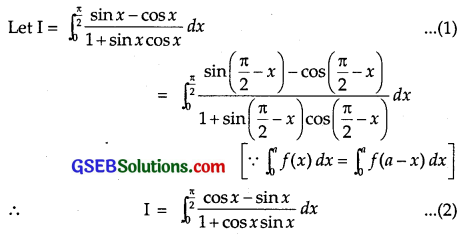Adding (1) and (2), we get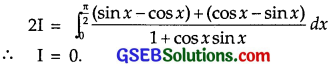Question 16.
$$\int_{0}^{π}$$log(1 + cosx)dx
Solution: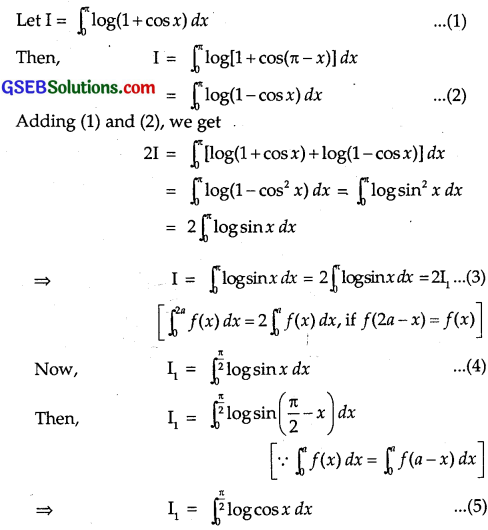Adding (4) and (5), we get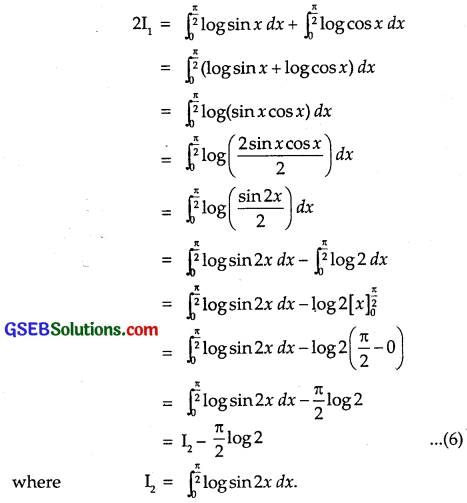Put 2x = t so that 2 dx = dt.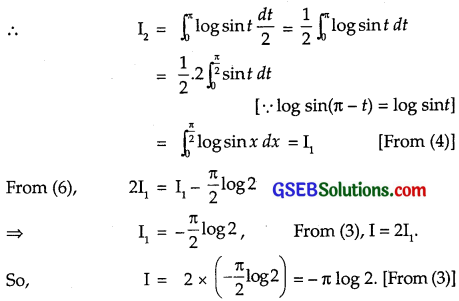Question 17.
$$\int_{0}^{a} \frac{\sqrt{x}}{\sqrt{x}+\sqrt{a-x}}$$dx
Solution: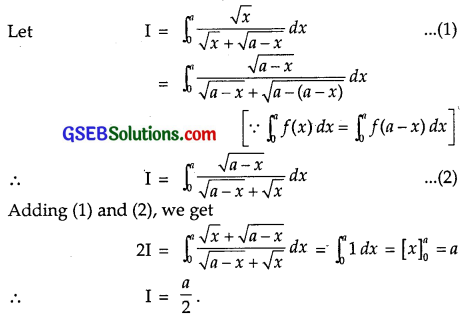Question 18.
$$\int_{0}^{1}$$|x – 1|dx
Solution: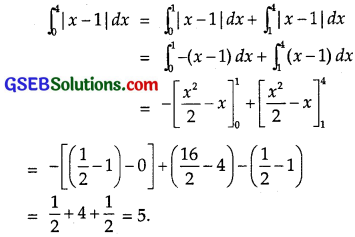Question 19.
Prove that $$\int_{0}^{a}$$ f(x)g(x) dx = 2$$\int_{0}^{a}$$ f(x)dx,
if f and g are defined as f(x) = f(a – x) and g(x) + g(x – a) = 4.
Solution: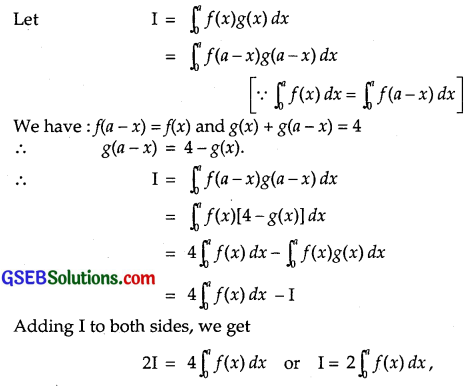Hence, the result.

Choose the correct answers in questions 20 and 21:
Question 20.
The value of $$\int_{-\frac{\pi}{2}}^{\frac{\pi}{2}}$$(x3 + x cosx + tan5x + 1) dx is
(A) 0
(B) 2
(C) π
(D) 1
Solution: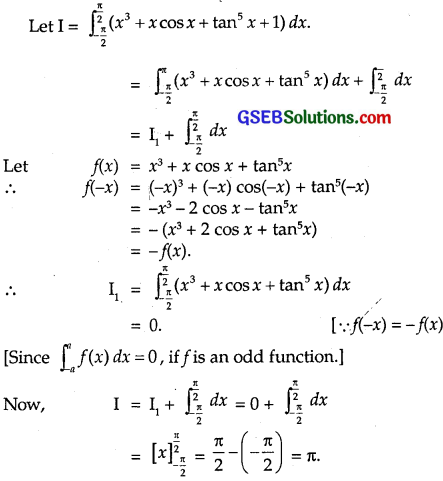∴ Part(C) is the correct answer.Question 21.
The value of $$\int_{0}^{\frac{\pi}{2}}$$ log ($$\frac{4+3sinx}{4+3cosx}$$) dx is
(A) 2
(B) $$\frac{3}{4}$$
(C) 0
(D) – 2
Solution: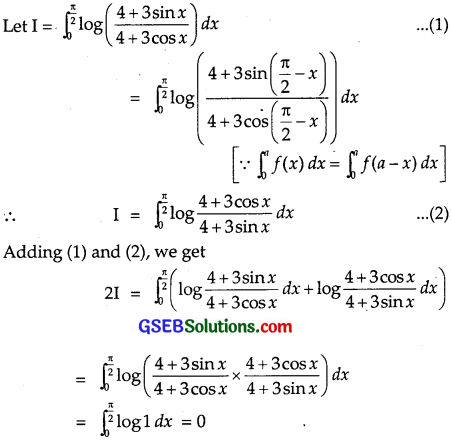∴ I = 0.
∴ Part(C) is the correct answer.